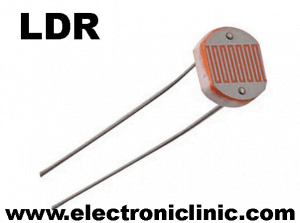# how to split a string message and access the sensors values

(Last Updated On: February 5, 2022)

## Split a String Message, Description:split a string message- In this tutorial, You learn how you can send multiple values from Arduino to Visual Basic application in one message and then how to split a string message and access each value separately. In this project, we will be using a comma”, ” as the delimiter.

To explain this we will be making An application in vb.net ” visual basic .net ” to receive a message and then split the message using the split function.

This tutorial will really help you in designing advanced level projects, in which multiple sensors are monitored. Read this article or watch Video given at the end of this article from start to the very end to learn how you can split a string and access the sensors values sent from the Arduino to visual basic application using Serial communication. The programming languages used are

1. visual basic 2010 express edition and
2. C language Arduino Uno IDE.

The Arduino programming is done in c language. This tutorial will cover the application designing, complete circuit diagram explanation, LDR and variable resistor interfacing, controller programming, and testing.

without any further delay, let’s get started!!!

Arduino Uno

Arduino Nano

Mega 2560:

LDR “light dependent resistor”:

Other Tools and Components:

Top Arduino Sensors:

Super Starter kit for Beginners

Digital Oscilloscopes

Variable Supply

Digital Multimeter

Soldering iron kits

PCB small portable drill machinesLDR stands for “LIGHT DEPENDENT RESISTOR”. LDR is basically a resistor whose resistance changes with the amount of light falling on the LDR. As the light intensity increases the resistance decreases. While with the decrease in light intensity the resistance increases. So we can say that an LDR is a variable resistor, whose resistance is controlled by the light.

The Basic working principle of the LDR is just like the ordinary resistor. Unlike the other resistors the LDR also has no polarity, so it doesn’t matter how you connect the two legs of the LDR. In this project, the Variable resistor will be used in series with another 10k resistor. So the LDR and the 10k resistor will make a voltage divider. The voltage on the middle of the LDR and 10k resistor will change as the light changes.

The output of the voltage divider circuit will be connected with the analog pin of the Arduino or Mega. Then using the Arduino programming we read the value of the LDR and combine this value with the value of the variable resistor and then send the complete message to the computer application, where these values will be split and displayed in desired boxes.

### Programming:

#### Arduino Programming:

The textForSMS variable which is of the type String will be used for making a complete message consisting of the LDR and variable resistor values separated by comma. Then I defined another variable of the type integer “ldr” and assigned it to the analog pin A1 of the Arduino. now we will refer to the analog pin A1 as the ldr. From now on ldr will be the name of the A1. Similarly defined pin A2 and assigned a name vresistor. Then I defined two variables of the type integer ldata and vdata.

As you know my friends every Arduino and Mega Program has at least two functions which are the void step and void loop functions. Void means that these functions are not returning any values, while the empty parenthesis means that these functions are not taking any arguments as the input. To establish the serial communication, I activated the serial communication using the Serial.begin() function and set the baud rate to 9600. This is the communication speed. Then I set the ldr and variable resistor as the input. One more thing about the void setup function is that, it executes only one time when the arduino or mega board is powered up.

In the void loop we read both the sensors “ LDR and vresistor “ and store the values in variables ldata and vdata. Then we add both the values with comma’s as the separators and store this in variable textForSMS. Then using the serial.println() function we send this message to the computer application. Finally we empty the string and use a delay of 1000 milli seconds which is equal to one second.  The comma is used as the delimiter which will be used to split a string message sent to the computer application.